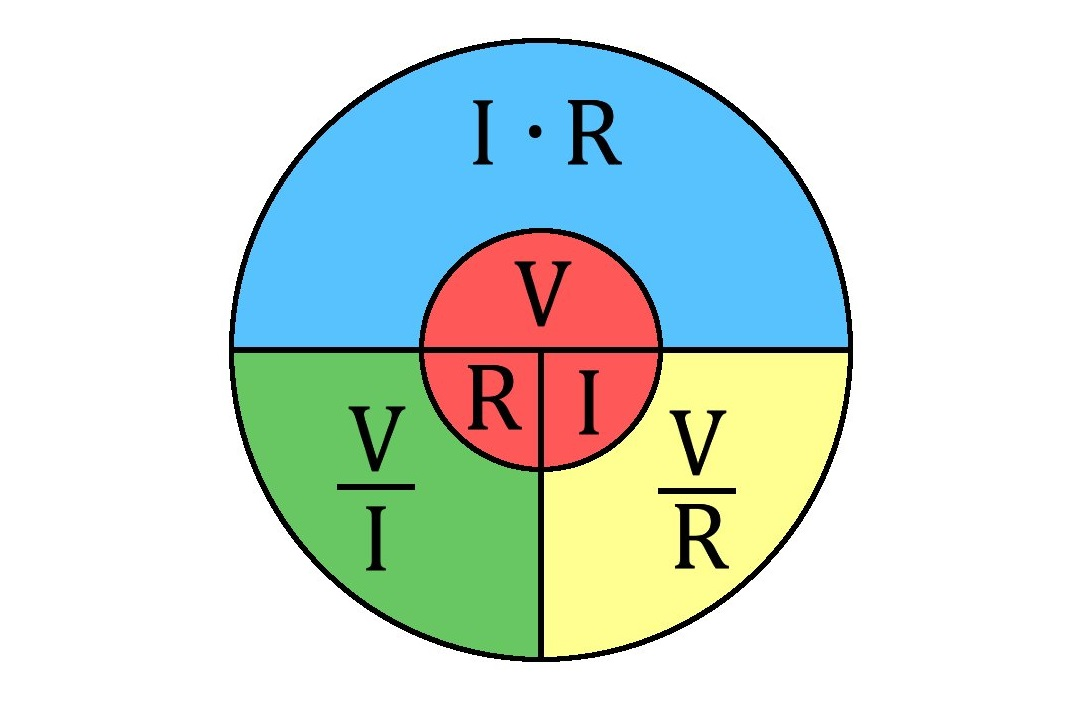# Ohm's Law Calculator

## How to Calculate Ohm's Law

Don’t be overwhelmed by the complexity of Ohm’s Law when it comes to calculating voltage, current or resistance – here’s a helping hand from Electrical Safety First.

## What is Ohm’s Law?

The principle behind Ohm’s Law – which is named after the Bavarian mathematician and physicist Georg Ohm, the man who first came up with it - in 1827.

It consists of three mathematical equations that explain the relationship between voltage, current and resistance. If you know two of these values, you can calculate the third using Ohm’s Law.

The law is usually applied to DC (direct current) circuits but can also be applied to many AC (alternating current) circuits if they are mainly resistive.

## How does Ohm’s Law work?

Ohm’s Law is actually very simple to apply and use.

Where V is voltage measured in volts, I is current measured in amperes, and R is resistance measured in ohms (sometimes represented by the Greek symbol Ω):

I x R = V (current multiplied by resistance = voltage)

V ÷ I = R (voltage divided by current = resistance)

V ÷ R = I (voltage Divided by resistance = current)

Here’s a useful visual reminder of how the formulas stack up:So for example, if you knew that the current of a circuit was 2 amperes and the resistant was 1 ohm, you could apply the following calculation to find out the voltage:

2 amperes x 1 ohm = 2 volts

If you knew that the voltage of a circuit was 2 volts and the current was 2 amperes, you could find out the resistance like this:

2 volts ÷ 2 amperes = 1 ohm

And if you knew the voltage was 2 volts and the resistance was 1 ohm, you’d calculate the current by doing this:

2 volts ÷ 1 ohm – 2 amperes

## Voltage, current and resistance explained

So what are the component parts that make up to Ohm’s Law?

Voltage is the pressure from a power source (for example, a battery) that pushes current through a circuit, while current is defined as the flow of electrons through a conductor.

Resistance is the opposition that a conductor (wire) offers to the current flow. As a general rule, resistance increases the longer the wire is, and decreases the thicker it is.

Each of these values can affect the others. For example, if there is too much resistance in a circuit, the voltage will drop or the current won’t flow as well.

Image sources:

https://commons.wikimedia.org/wiki/File:Ley_de_ohm_-_Organigrama.jpg